# Tank Experiment¶

For this analysis, we'll start by making a standard curve from a laboratory data and then use this with residence time distribution theory to determine the residence times and the equalibrium concentration based on annual dust deposition.

### Steps¶

2. Fit curve of $C(t) = Y - \frac{At}{t+K}$
3. Generate residence time distribution data
4. Run diffusion-scavenging model
5. Compare residence time distributions between idealized diffusion data and (3).
6. Add settling velocities to diffusion model

## Standard Curve¶

The Standard Curve is based on a laboratory bomb-style experiment where trace element concentrations were recorded following an initial large-deposition event. Both particulate and dissolved forms were measured.

The model used was inspired by enzyme kinetics and allows for a residual concentration to remain as $t \to \inf$ (most functional responses assume that C would return to 0 or inital conditions.).*

$$C(t) = Y - \frac{At}{t+K}$$

Here $Y$ is the initial concentration after the depositional event, $A$ is a scale factor that controls the residual concentration as $t\to\inf$, and $K$ controls the speed, or velocity, of decay.

To fit the model, we used a iterative approach using the Sum Squared Residuals (SSR) as a cost function. With each sampling iteration, a set of $A$ and $K$ were fit to a randomly sampled set (with replacement) of data. The quality of the resulting fit was determined (via SSR).

Once the fits were made, the parameters were saved and the resulting $R^2$ values were computed:

$$R^2 = 1 - SSR/SST$$

where $SSR = \sum{(C(t) - x_i)^2}$ and $SST = \sum{x_i-\bar{x}}$.

#### data.s¶

In this section, the original data is scaled for use in the fitting function (to normalize the paramaters used to fit the function inside the model() function. For conveiance, concentrations are converted to percentage of the inital value.

*Other functional fits were tested including exponential and power but they resulting in a poor fit. Additionally, this model maintains just three fit parameters (Y, A and K), the minimum degrees of freedom required in order to allow a residual concentration (i.e. residual, slope, intercept).

In :
## Load the data from csv
data = read.csv("~/Dropbox/Documents/Alina_Tank Concentrations V4.csv")

## Clean up the data structure and move time to own variable.
data = data[-1,]
Time = data$Time*60-60 # minutes from first sampling point data = data[,-1] * 1e3 # ug/L --> ug/m3 print('Raw Data') head(data) ## Scaled 0->100% data for use in fitting the model. data.s = data for (i in 1:ncol(data)) { data.s[,i] = data[,i]/max(data[,i]) * 100 } print('Scaled data') head(data.s)   "Raw Data"  dAldMndFedCodNidCudZndYdCddPbtpAltpMntpFetpCotpNitpCutpZntpYtpCdtpPb 213509.196252873 3570.133428236 8436.179397314 80.195944685 176.815969465 246.71474317 881.017835581 66.684565 29.965215061 26.044948359 178875.783121035762.008327449 100595.57167649235.24607452 267.771403058 72.085978685 410.843546187 40.48926529 3.798844767 47.640901191 32595.389188777 2656.060435003 761.472743103 60.512534861 177.772853029 226.178941388 504.172403161 67.635075109 25.542847058 20.48546293 134663.7546315091112.279968129 77056.992644655 41.01483369 99.188921333 96.490908765 259.068839295 39.784797822 1.648424846 59.174876735 42026.214387581 939.171219011 441.368113116 40.947936778 195.711845122 196.799536524 371.390684128 59.939092474 18.14634777 21.059004539 77108.751980888636.043117076 40925.93756262423.79461327 80.160600269 33.133033792 211.461324629 22.302015433 1.5221856 45.369617885 52035.701075204 674.713158878 473.725514987 37.807571625 193.683618088 199.939429693 343.09591183 65.464617466 18.013056281 30.975266613 44608.798881135316.140375038 25863.37200904213.301364276 33.786778317 20.203103447 169.538731029 9.194621899 0.436791277 14.252386853 61292.578236862 1093.481125263 324.798772875 33.715917826 193.003465434 192.632961812 289.690737173 52.602364242 15.071180991 17.830463974 43103.409780501358.335843564 24164.75320549313.595357278 29.293399063 15.503739104 146.74516936 13.258736323 0.451076531 19.589350775 71172.638301812 704.10104097 205.810007906 27.184848403 193.551995451 197.097958743 315.098982833 39.424799258 12.956869286 17.806405797 52103.112859228434.651343076 28596.28470616116.834082507 41.635890957 23.952484707 227.168518253 15.864420851 0.64160751 35.448604474  "Scaled data"  dAldMndFedCodNidCudZndYdCddPbtpAltpMntpFetpCotpNitpCutpZntpYtpCdtpPb 2100 100 100 100 83.9797352283807100 100 98.5946491410438100 84.0830482087576100 68.5086803038265100 85.9349443823138100 74.7075342202059100 100 100 80.5086614786676 319.212017800282174.39667139598649.0262748957833475.455853907184484.434212437607191.676297282384357.2261290066297100 85.241661059340366.134904296198975.2833906758579100 76.6007800944406100 37.0423877233505100 63.057784818428798.260112988086343.3927929964294100 414.998778237084926.306333863691 5.2318483560997751.059859621129992.954437227214479.768048717053942.154729351543888.621314277248560.558042827523867.986515829251243.107429432587357.183724898499 40.683637341650458.014652576298229.936206537946534.337985014416551.470036852605455.081304324156640.069697325434376.6704053954799 515.069002160443618.89882191913975.6154035218576547.143994342237191.991119435197581.040730328479338.943128955356596.790928908555 60.1132220954561100 24.938422687967128.422733852681825.710249047758 32.430618581889 12.617769459751320.937831040853741.266008095410722.708789189293811.498002782170524.0851990563931 69.5681357548352130.62857865800523.8500695347755742.041923638947291.668077121934578.079225966348232.881370327986577.773794376995350.295587601556357.563552871944424.096839174331 32.216335260156524.021686842443833.147415349180710.939704064162116.067564605240535.718017411233132.746300107042511.874044839063633.1041682819654 78.6802965910175119.72198112824862.4396115612658533.898033759411291.928604526398479.889007122362735.765335286907558.290464222096943.239700631628357.485883881066729.128097694461439.077512454633228.426981654942641.043888253298915.549043132130724.823566296111355.293193810960339.181794822338216.889542725555759.9048218262419 In : ## Load dust flux data, convert to minutes Dust = read.csv('~/Dropbox/Documents/Alina_Dust Fluxes Combined.csv') #ug m-2 min-1 Dust = Dust[,-1] str(Dust)  'data.frame': 4 obs. of 40 variables:$ dAl : num  1.00 1.00 1.92e-05 1.92e-06
$dMn : num 1.00 1.00 2.49e-06 2.49e-07$ dFe : num  1.00 1.00 1.01e-05 1.01e-06
$dCo : num 1.00 1.00 1.71e-08 1.71e-09$ dNi : num  1.0 1.0 1.9e-07 1.9e-08
$dCu : num 1.00 1.00 2.47e-07 2.47e-08$ dZn : num  1.00 1.00 2.55e-06 2.55e-07
$dY : num 1.00 1.00 2.09e-08 2.09e-09$ dCd : num  1.00 1.00 5.71e-08 5.71e-09
$dPb : num 1.00 1.00 1.33e-08 1.33e-09$ lAl : num  1 1 0.003927 0.000393
$lMn : num 1 1 0.001889 0.000189$ lFe : num  1 1 0.002793 0.000279
$lCo : num 1.00 1.00 1.41e-05 1.41e-06$ lNi : num  1.00 1.00 1.17e-05 1.17e-06
$lCu : num 1.0 1.0 1.9e-05 1.9e-06$ lZn : num  1.0 1.0 3.5e-05 3.5e-06
$lY : num 1.00 1.00 4.83e-05 4.83e-06$ lCd : num  1.00 1.00 1.64e-06 1.64e-07
$lPb : num 1.00 1.00 4.19e-05 4.19e-06$ rAl : num  1 1 0.7038 0.0704
$rMn : num 1 1 0.002942 0.000294$ rFe : num  1 1 0.3187 0.0319
$rNi : num 1.00 1.00 9.63e-05 9.63e-06$ rCo : num  1.00 1.00 1.94e-04 1.94e-05
$rCu : num 1.00 1.00 2.55e-04 2.55e-05$ rZn : num  1.00 1.00 5.86e-04 5.86e-05
$rY : num 1.00 1.00 1.57e-04 1.57e-05$ rCd : num  1.0 1.0 1.9e-08 1.9e-09
$rPb : num 1.00 1.00 1.08e-04 1.08e-05$ tpAl: num  1 1 0.7078 0.0708
$tpMn: num 1 1 0.004833 0.000483$ tpFe: num  1 1 0.3215 0.0322
$tpCo: num 1.0 1.0 1.1e-04 1.1e-05$ tpNi: num  1.00 1.00 2.05e-04 2.05e-05
$tpCu: num 1.00 1.00 2.74e-04 2.74e-05$ tpZn: num  1.00 1.00 6.24e-04 6.24e-05
$tpY : num 1.00 1.00 2.05e-04 2.05e-05$ tpCd: num  1.00 1.00 1.71e-06 1.71e-07
$tpPb: num 1.0 1.0 1.5e-04 1.5e-05  ### Fitting Function¶ This code block is used to determine the quality of the fit (or cost) which is then optimized. The first function, fit, calculates the sum-of-squares error associated with the second function, fitted, and the data, d. The current functional form is:$f = params - \frac{params \cdot t}{t+params}$In : ## Helper Functions fit = function(p, t, d) { dif = fitted(p, p, p, t) - d sum(dif^2) } fitted = function(Y, A, K, t) { {Y-A*t/{t+K}} } fitted.p = function(p, t) { {p-p*t/{t+p}} }  ### Model function¶ The model() function is used to generate a fit for the data using the function fit() to determine the quality. The model is provided the data and the index j which is the column within dat to fit. This model uses a normally distributed relative error adjustment to the original data since this result is used for bootstrap the variance (i.e. we allow the concentrations to vary with stdev of 10%). Once an inital fit is calcualted (i.e. K = 1), the loop then iteratively adjusts the fit using a gradient descent technique. For this model, Y, A and K are all adjusted. In : model = function(j, data.s) { d = data.s[,j] dd = rnorm(length(d), d, d*0.1) ## Inital Guess A = 80 K = 100 Y = max(dd) #Fit model (nonlinear gradient method) res = nlm(fit, c(Y, A, K), Time, dd, stepmax=10) # New params Y = res$estimate
A = res$estimate K = res$estimate
return(data.frame(j,colnames(data.s)[j], Y, A, K, res$minimum)) }  The model is then run for n times for each of the elements and the results are saved in the well-named variable temp. In : temp = model(1, data.s) n = 100 for (j in 1:ncol(data.s)) { for (i in 1:n) { temp = rbind(temp, model(j, data.s)) } } for (i in 1:ncol(data.s)) { l = temp$j==i
temp$A[l] = temp$A[l]/100.0 * max(data[,i])
temp$Y[l] = temp$Y[l]/100.0 * max(data[,i])
}
str(temp)

'data.frame':	4001 obs. of  6 variables:
$j : num 1 1 1 1 1 1 1 1 1 1 ...$ colnames.data.s..j.: Factor w/ 40 levels "dAl","dMn","dFe",..: 1 1 1 1 1 1 1 1 1 1 ...
$Y : num 10282 9301 10695 9276 12182 ...$ A                  : num  10114 8404 10163 8140 10550 ...
$K : num 26 18 43 21.8 8.9 ...$ res.minimum        : num  527 1132 2110 1118 770 ...


### Plot the data interpolation fits¶

In :
# Optional output plotting the Fit lines with the data.
pdf('~/temp/Alina_Fits.pdf')
par(mfrow=c(2,2))
#if (0) {
for (j in 1:ncol(data)) {
l = (temp$j == j) plot(Time, data[,j], xlab='Time (hr)', ylab=colnames(data)[j]) for (i in c(1:nrow(temp))) { if (l[i]) { lines(Time, fitted(temp$Y[i], temp$A[i], temp$K[i], Time), col='#FF000003')
}
}
}
dev.off()

png: 2

### Calculate R^2 and save dust flux¶

In :
temp$R2 = 0 ## Add Dust fluxes to models temp$MJMin = 0 # ug m-2 min-1
temp$MJAvg = 0 temp$MJMax = 0

temp$SJMin = 0 temp$SJAvg = 0
temp$SJMax = 0 for (i in 1:nrow(temp)) { k = temp$j[i]
temp$R2[i] = 1-temp$res.minimum[i]/sum((data[,k]-mean(data[,k]))^2)

## Moroccan
temp$MJMin[i] = as.numeric( Dust[3, k] - Dust[4, k] ) temp$MJAvg[i] = as.numeric( Dust[3, k] )
temp$MJMax[i] = as.numeric( Dust[3, k] + Dust[4, k] ) ## SW temp$SJMin[i] = as.numeric( Dust[1, k] - Dust[2, k] )
temp$SJAvg[i] = as.numeric( Dust[1, k] ) temp$SJMax[i] = as.numeric( Dust[1, k] + Dust[2, k] )
}

str(temp)
#write.csv(ans, '~/Desktop/R2.csv')

'data.frame':	4001 obs. of  13 variables:
$j : num 1 1 1 1 1 1 1 1 1 1 ...$ colnames.data.s..j.: Factor w/ 40 levels "dAl","dMn","dFe",..: 1 1 1 1 1 1 1 1 1 1 ...
$Y : num 10282 9301 10695 9276 12182 ...$ A                  : num  10114 8404 10163 8140 10550 ...
$K : num 26 18 43 21.8 8.9 ...$ res.minimum        : num  527 1132 2110 1118 770 ...
$R2 : num 1 1 1 1 1 ...$ MJMin              : num  1.73e-05 1.73e-05 1.73e-05 1.73e-05 1.73e-05 ...
$MJAvg : num 1.92e-05 1.92e-05 1.92e-05 1.92e-05 1.92e-05 ...$ MJMax              : num  2.11e-05 2.11e-05 2.11e-05 2.11e-05 2.11e-05 ...
$SJMin : num 0 0 0 0 0 0 0 0 0 0 ...$ SJAvg              : num  1 1 1 1 1 1 1 1 1 1 ...
$SJMax : num 2 2 2 2 2 2 2 2 2 2 ...  # Residence time distribution¶ Based on RTD theory, residence time can be calculated as follows which yields a curve of the proportion of particles of a particular residence time.$E(t) = \frac{C(t)}{\int^\infty_0C(t)dt}$For our discrete data then,$E(t) = \frac{C(t)}{\sum C(t)\Delta t}$From this function, the residence time distribution, the residence time can be calculated by this formula and is simply the area under the curve defining the residence time relative abundance (its a bit complicated, but a simple explaination is possible upon request):$\tau = \int^\infty_0t\cdot E(t)dt = \sum (t \cdot E(t)\Delta t)$In : ## Calculate E(t) and t*E(t) for each model fit t = seq(0,24*100,1) temp$ETau = 0

for (i in 1:nrow(temp)) {
s = sum((temp$A[i] - temp$A[i] * t/(t+temp$K[i]))) # E(t) is: e = (temp$A[i] - temp$A[i] * t/(t+temp$K[i])) / s

#save value in temp
temp$ETau[i] = sum(t * e) }  In : ## Let's calculate the resulting average residence time for each element: m = c() s = c() for (i in 1:ncol(data)) { m = c(m, mean(temp$ETau[temp$j == i])) s = c(s, sd(temp$ETau[temp$j == i])) } data.frame(n=c(1:ncol(data)), m/60, s/60)  nm.60s.60 11.00000007.99896830.9018921 22.00000009.94038720.3110008 33.0000008.2631601.233669 4 4.000000010.5691411 0.4750978 5 5.0000000010.65210935 0.03216732 66.0000009.8428741.137977 77.00000009.26006520.5815939 8 8.000000011.9153428 0.2270654 9 9.000000011.3830948 0.5044682 1010.000000010.2440864 0.7957841 1111.000000011.0341793 0.3079131 1212.000000012.0316023 0.2024191 1313.000000010.8333788 0.4027798 1414.00000011.869226 0.283931 1515.000000 8.228055 1.188457 1616.0000000 8.0261159 0.6514588 1717.00000010.225996 0.975432 1818.000000011.8135962 0.2615651 1919.000000 8.629460 1.187406 2020.000000011.9881661 0.2626207 2121.000000010.8871133 0.3893169 2222.000000011.4726069 0.3249513 2323.000000010.8285844 0.3860856 2424.000000011.7788337 0.2446745 2525.000000010.1819911 0.3899635 2626.000000011.7592152 0.1192481 2727.000000011.5968240 0.3313256 2828.000000011.1349005 0.3888132 2929.000000011.7482946 0.1128297 3030.000000011.1508871 0.4397593 3131.000000010.8819224 0.4149682 3232.000000011.9328751 0.2812431 3333.000000010.7955347 0.3561657 3434.000000011.9437398 0.1361882 3535.000000 8.985376 0.325858 3636.000000011.1336845 0.2300291 3737.000000011.3464137 0.4554302 3838.000000011.6297813 0.2879301 3939.0000000 8.2116418 0.4043223 4040.000000011.8930047 0.2076678 # Diffusivity Analysis to compare tau between measured concentration and theoretical¶ Calculate sml concentration profile over time: 1. Initial freshwater lens profile using a pulse of 1 yr of dust 2. Allow diffusion to erode profile saving the sml concentration at each minute. Use results from above to calculate Eq and Tau 1. Initialize Ci with 1 yr of dust flux 2. Calculate E(t) as above 3. Calculate tau and compare to tau from above In : ## initialize setup library(compiler) enableJIT(3) w = 47e-6 ## 47 um d = seq(0,25, 0.1) ## Depth domain (100 micro resolution) dz = d - d k2 = 1e-3/60 # per minute rr = 60*100 # number of minutes to run model for  3 In : ######################################### ##~~~ Calculate Diffusion Profile ~~~~ ## ######################################### dprofile = data.frame(matrix(0, nrow=length(d), ncol=10)) dhistory = data.frame(matrix(0, ncol=10, nrow=rr)) f = 2 end = length(d) dt = 1.5/f # s dust = Dust[3,1:10] * 60 * 24 * 30 * 12 # dust flux per year dprofile[which(d < 5.6),] = t(dust) / 5.6e-3 D = f*as.numeric(DiffusionCoefficients[1:10] * 1e6) ## mm^2/s for (i in 1:(60)) { ## each loop is a minute for (j in 1:(60/dt)) { ## Loop for every minute (each k is a dt second) for (k in 1:ncol(dprofile)) { #for (k in 1:2) { d2C = dprofile[c(2:end,end), k] + dprofile[c(1,1:(end-1)), k] - 2 * dprofile[1:end,k] d2C = as.numeric(d2C) dprofile[,k] = dprofile[,k] + D[k] * (d2C/dz^2) * dt - dprofile[,k] * k2 * dt# } } } dt = 2 * dt ll = which(d < .047) for (i in 1:rr) { ## each loop is a minute for (j in 1:(60/dt)) { ## Loop for every minute (each k is a dt second) for (k in 1:ncol(dprofile)) { #for (k in 1:2) { d2C = dprofile[c(2:end,end), k] + dprofile[c(1,1:(end-1)), k] - 2 * dprofile[1:end,k] d2C = as.numeric(d2C) dprofile[,k] = dprofile[,k] + D[k] * (d2C/dz^2) * dt - dprofile[,k] * k2 * dt# } } dhistory[i,] = sapply(dprofile[ll,], mean) }  In : #pdf('~/Desktop/RTD-k-3.pdf') par(mfrow=c(2,2)) for(i in 1:10) { plot(c(1:(nrow(dhistory)))*dhistory[,i]/sum(dhistory[,i]), type='l', main=colnames(data)[i], xlab='Time (min)', ylab='t*E(t)') tt = data[,i]/1e3 tt = (tt-min(tt)) / (1 - min(tt)/max(tt)) T = Time - c(60,Time[1:(length(Time)-1)]) points(Time, Time * tt/sum(tt * T), col='#ff000050') text(5000,.25, paste('Data Tau =', format(sum(Time * tt/sum(tt * T) * T)/60,digits=3), 'hr'), cex=0.6, col='#ff000080') text(5000,0.27, paste('Diffusion Tau =', format(sum(c(61:(nrow(dhistory)+60))*dhistory[,i]/sum(dhistory[,i]))/60, digits=3), 'hr'), cex=0.6) #text(5000,0.29, paste('Fit Tau =', format(m[i]/60,digits=3), 'hr'), cex=0.6, col='blue') l = temp$j == i
tim = c(1:6e3)
A = mean(temp$A[l]) K = mean(temp$K[l])
Y = mean(temp$Y[l]) s = sum((A - A * tim/(tim+K))) # E(t) is: e = (A - A * tim/(tim+K)) / s -3.5e-5 lines(tim, e*tim, cex=0.6, pch=4, col='red') text(5000,0.29, paste('Fit Tau =', format(sum(e*tim)/60,digits=3), 'hr'), cex=0.6, col='red') } #dev.off()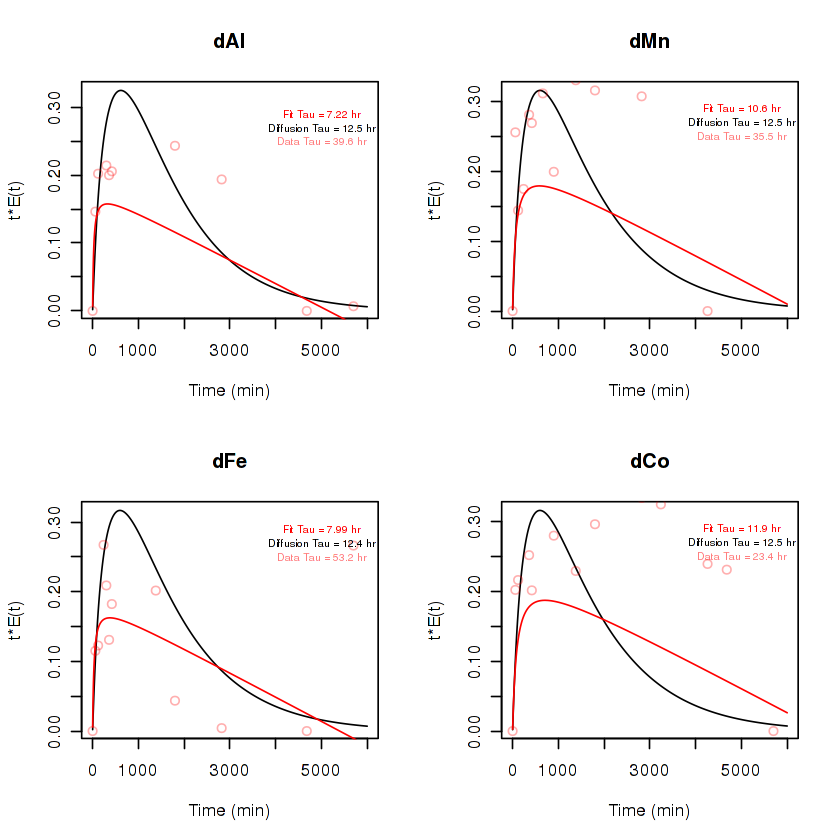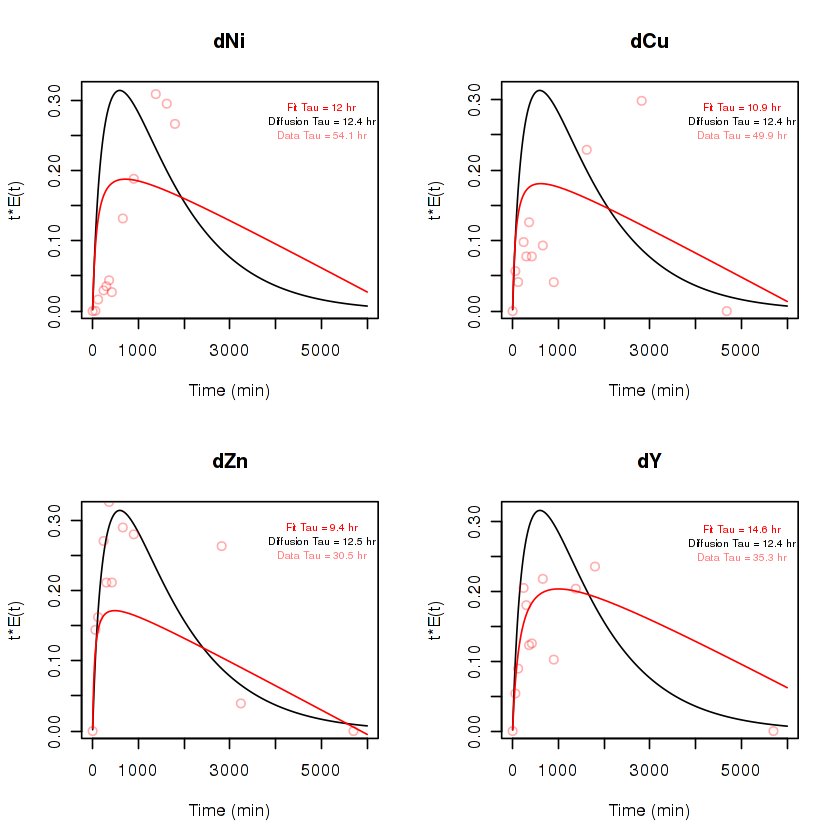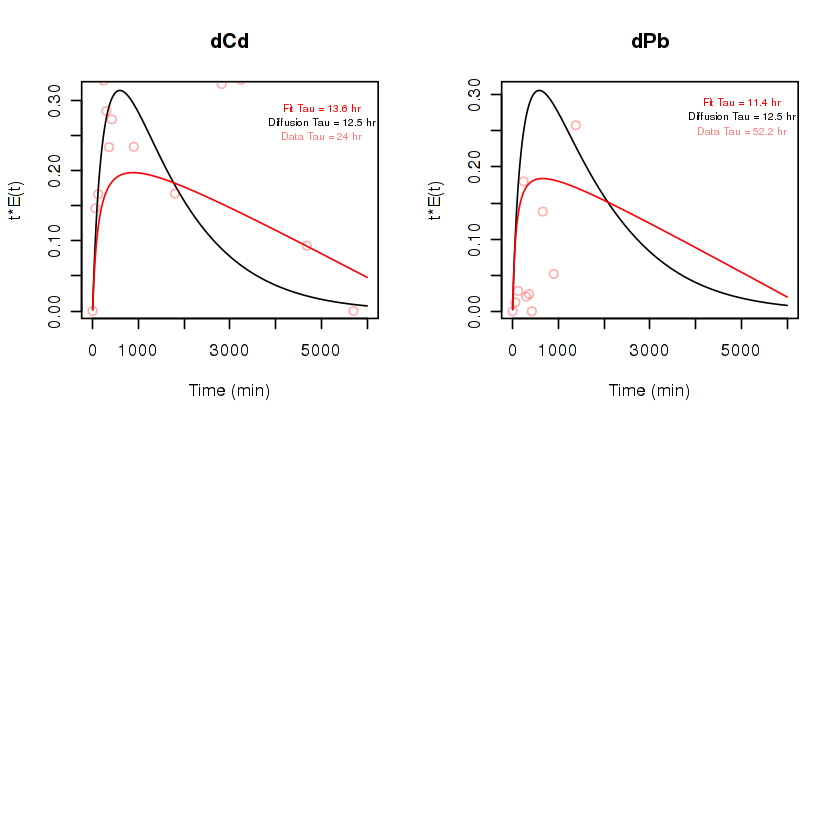In :   684.558125372663 In : save(dhistory, file='~/Dropbox/Documents/Alina_dhistory2.rdata') #load('~/Dropbox/Documents/Alina_dhistory.rdata')  In [ ]: #pdf('~/Desktop/Alina_Data versus diffusion model (rel and abs).pdf') par(mfrow=c(2,2)) for(n in 1:10) { tt = data.s[,n]/100 tt = (tt-min(tt)) / (1 - min(tt)/max(tt)) plot(Time, tt, xlim=c(0,1500), ylim=c(0,1), main=colnames(data)[n], xlab="Time (min)", ylab="Rel Conc.") lines(c(1:nrow(dhistory))-60, dhistory[,n]/dhistory[60,n], pch=2, col='#FF0000') #legend(1000, 1, c("k = 1e-2", "k = 1e-3"), col=c('#FF0000','#FF00F0'), lwd=3, cex=0.6) tt = data[,n]/1e3 tt = (tt-min(tt)) / (1 - min(tt)/max(tt)) plot(Time, tt, xlim=c(0,1500),ylim=c(0,max(tt)*1.25), main=colnames(data)[n], xlab="Time (min)", ylab="Conc. (ug/L)") lines(c(1:nrow(dhistory))-60, dhistory[,n], pch=2, col='#FF0000') #legend(1000, max(tt)*1.1, c("k = 1e-2", "k = 1e-3"), col=c('#FF0000','#FF00F0'), lwd=3, cex=0.6) } #dev.off()  ## Dissolved done, not it's time for particles¶ Steps 1. load particle information 2. run diffusion model for them with same k (opposite sign) 3. keep track of relative enrichment of TE on particles 4. run RTD analysis as above In : ## load and prep information about particles particles = read.csv('~/Dropbox/Documents/Alina_Tank Model Size Spectrum.csv') particles$k = k2 * particles$SA.m2. / sum(particles$SA.m2.)
particles$m = particles$D.µm.^3 * 4*pi/3 * 1e-18
particles$m = particles$m/sum(particles$m) particles$v.ms = particles$v.ums * 1e-6 profile = data.frame(matrix(0,ncol = nrow(particles), nrow=length(d)))## Current vertical profiles history = data.frame(matrix(0, ncol=nrow(particles), nrow=rr))## the sml concentrations of each  In : ## Prep the workspace for the particle analysis m = 1 # which TE end = length(d) dt = 1.5/f # s dust = Dust[3,m+20] * 60 * 24 * 30 * 12 * particles$m
profile[1,] = dust / 5.6e-3
D = f*as.numeric(DiffusionCoefficients[m] * 1e6) ## mm^2/s

In :
e = rep(1, ncol(profile)) # enrichment factor

#########################################
##~~~ Calculate Diffusion Profile ~~~~ ##
#########################################
for (i in 1:(rr)) { ## each loop is a minute
#Cd = dhistory[nrow(history), m]

for (j in 1:(60/dt)) { ## Loop for every minute (each k is a dt second)
for (k in 1:ncol(profile)) { # each particle
d2C = profile[c(2:end,end),k] + profile[c(1,1:(end-1)),k] - 2 * profile[,k]
## Diffusion
profile[,k] = profile[,k] + D * (d2C/dz^2) * dt
## Scavenging
#e[k] = e[k] + (Cd * k2 * particles$k[k] * dt) / profile[1,k] ## Settling profile[,k] = profile[,k] * (1-particles$v.ms[k]/w * dt)
profile[profile[,k] < 0, k] = 0
}
}
history[i,] = profile[1,] * e
}

In :
#save(history, file=paste0('~/Dropbox/Documents/Alina_history-',colnames(data)[n] , '.rdata'))

In :
pdf(paste0('./Particulate Model Results-', colnames(data)[m], '.pdf'))
par(mfrow=c(2,2))

tt = data.s[,m+10]/100
tt = (tt-min(tt)) / (1 - min(tt)/max(tt))

plot(Time, tt, xlim=c(0,1500), ylim=c(0,1),
main=colnames(data)[m], xlab="Time (min)", ylab="Rel Conc.")

lines(c(1:nrow(history)), apply(history, 1, sum)/apply(history, 1, sum), pch=2, col='#FF0000')

for (i in 1:ncol(history)) {
lines(c(1:nrow(history)), history[,i]/apply(history, 1, sum), pch=2, col='#FF000020')
}

tt = data[,n+10]/1e3
tt = (tt-min(tt)) / (1 - min(tt)/max(tt))

plot(Time, tt, xlim=c(0,1500),ylim=c(0,max(tt)*1.25),
main=colnames(data)[m], xlab="Time (min)", ylab="Conc. (ug/L)")

lines(c(1:nrow(history)), apply(history, 1, sum), pch=2, col='#FF0000')

for (i in 1:ncol(history)) {
lines(c(1:nrow(history)), history[,i], pch=2, col='#FF000020')
}

dev.off()

png: 2

# Sulfate and Diffusion Animation¶

Calculate the observed diffusion coefficient from sulfate data.

Also make animations.

In :
# interpolate sulfate data onto modeldata$Time modeldata$sulfate1 = 0
modeldata$sulfate2 = 0 sulfate_d1 = .05 sulfate_d2 = 300 #30cm  timesmluwc 23600.00 12.68 23.95 37200.00 15.91 24.01 410800.00 18.42 23.96 518000.00 19.61 23.91 621600.00 20.00 25.07 743200.00 21.73 24.53 In : for(i in 1:nrow(modeldata)) { i1 = which.min(abs(sulf$time-modeldata$Time[i])) if ( sulf$time[i1] < modeldata$Time[i]) { i2 = i1 + 1 } else { if (i1 == 1) { i2 = 2 } else { i2 = i1 -1 } } m1 = (sulf$sml[i2] - sulf$sml[i1]) / (sulf$time[i2] - sulf$time[i1]) m2 = (sulf$uwc[i2] - sulf$uwc[i1]) / (sulf$time[i2] - sulf$time[i1]) modeldata$sulfate1[i] = m1 * (modeldata$Time[i] - sulf$time[i1]) + sulf$sml[i1] modeldata$sulfate2[i] = m2 * (modeldata$Time[i] - sulf$time[i1]) + sulf$uwc[i1] } summary(modeldata$sulfate1)

   Min. 1st Qu.  Median    Mean 3rd Qu.    Max.
9.45   20.00   21.73   20.31   21.84   21.95 
In :
i=380
plot(c(2:ncol(modeldata)*dz), 0.90 * (modeldata[i,2:ncol(modeldata)] - 25) + 25,
xlab="Depth (mm)", ylab="Concentration",
pch=4, cex=0.5,
ylim=c(0,25))

points(sulfate_d1, modeldata$sulfate1[i], col='blue') points(sulfate_d2, modeldata$sulfate2[i], col='blue')

text(100, 0.025, paste(format(modeldata$Time[i]/60, digits=3), 'minutes'))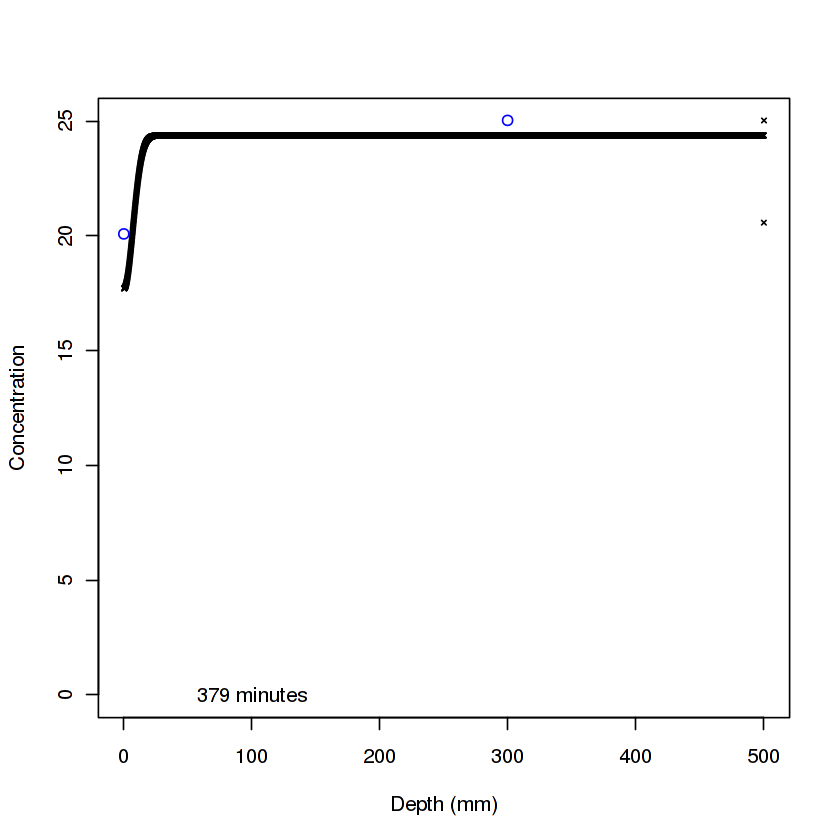In : for (i in 1:nrow(modeldata)) { # creating a name for each plot file with leading zeros if (i < 10) {name = paste('0000',i,'plot.png',sep='')} if (i < 100 && i >= 10) {name = paste('000',i,'plot.png', sep='')} if (i >= 100) {name = paste('00', i,'plot.png', sep='')} if (i >= 1000) {name = paste('0', i,'plot.png', sep='')} #saves the plot as a .png file in the working directory png(paste("./images/", name, sep='')) plot(c(2:ncol(modeldata)*dz), modeldata[i,2:ncol(modeldata)], xlab="Depth (mm)", ylab="Concentration", pch=4, cex=0.5, ylim=c(10,25)) points(sulfate_d1, modeldata$sulfate1[i]*1, col='blue')
points(sulfate_d2, modeldata$sulfate2[i]*1, col='blue') text(400, 15, paste(format(modeldata$Time[i]/60, digits=3), 'minutes'), cex=0.7)
dev.off()
}


## Misc output¶

Out of date and not relevent for anything but prosterity.

In :
for (i in 1:ncol(data)) {
write.csv(temp[temp$j == i,], paste0('~/temp/Model_', colnames(data)[i], '.csv')) }  In : summary = data.frame(Element=0, Y=0, Ys=0, A=0, As=0, K=0, Ks=0, Eq=0, Eqs=0, Tau=0, Taus=0) for (i in 1:ncol(data)) { l = (temp$j == i)

temps = temp[l,]
l = which(!is.na(temps$MEQ.avg)) summary = rbind(summary, c(colnames(data[i]), mean(temps$Y[l]), sd(temps$Y[l]), mean(temps$A[l]), sd(temps$A[l]), mean(temps$K[l]), sd(temps$K[l]), mean(temps$MEQ.avg[l]), sd(temps$MEQ.avg[l]), mean(temps$MTau.avg[l]), sd(temps\$MTau.avg[l]))
)
}
write.csv(summary, '~/temp/Hyperbolic Summary.csv')

In :
head(summary)

100000000000
2dAl 10500.95008155821290.622633098769477.149062765341154.3900617047422.893563611279614.4768128214413300.16877195572999.0348170977059656.067888581936216.45677172232
3dMn 3656.9977976607 331.5105967902943428.85843375944335.26940609881668.796165193011 11.7945545644649117.4182076101977.324741628492641978.64917300497123.431401829995
4dFe 5713.88528760273677.6718436077835610.07789892427721.58678319540431.444998391087420.8370589962678194.96217075985880.2594819346 807.472591361563332.409777786551
5dCo 81.7449372226806 7.53812055783378 64.3171139256435 7.3364996408763 92.7927114112627 24.7343199221966 1.51844014399505 0.1411843021521913724.42998519169 346.296856318951
6dNi 216.511413456139 7.37647885589541 15.445417831356 7.06932494013277 95.7584976806893 2.79120432625674 2.49528672995193 0.683005304617921550.839536210312 150.774787005005
In :
par(mfrow=c(3,3))

for (i in 1:ncol(data)) {
plot(Time, data[,i], ylim=c(0,max(data[,i])), main=colnames(data)[i])
}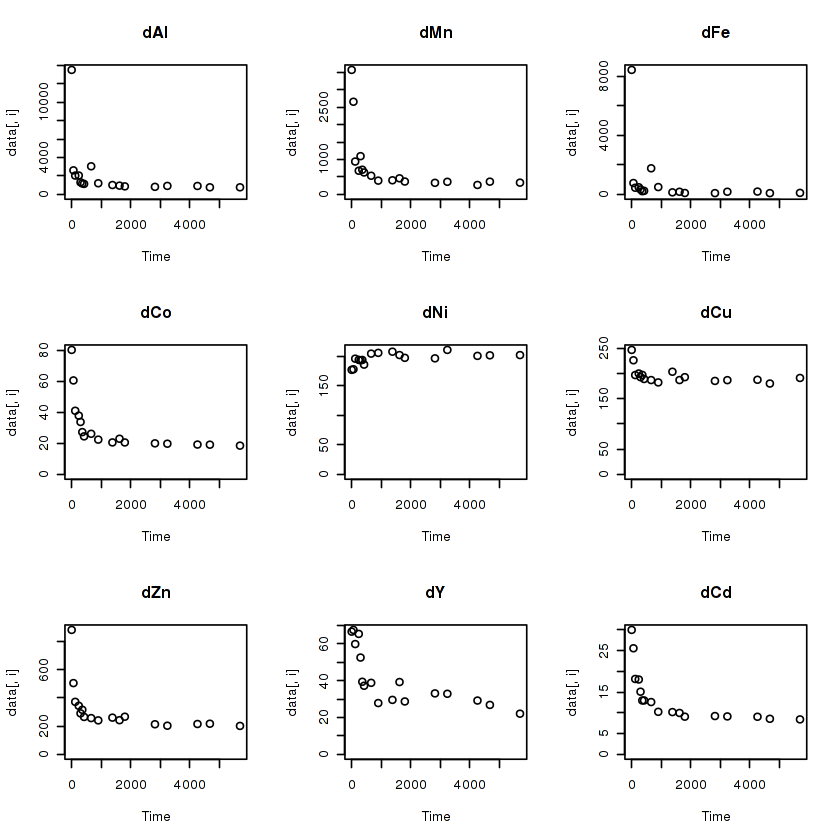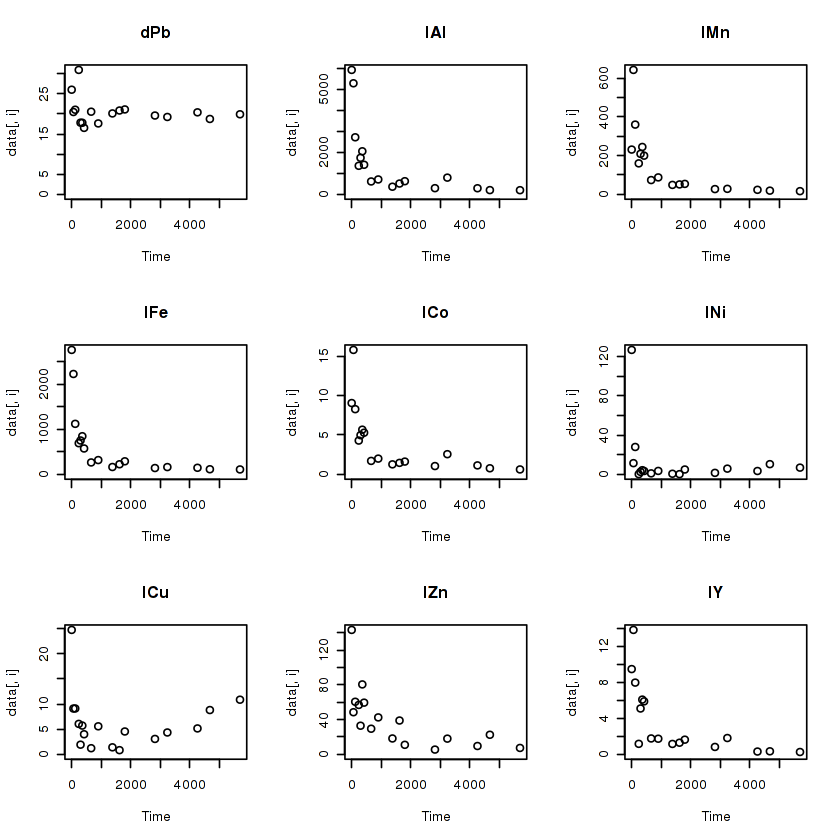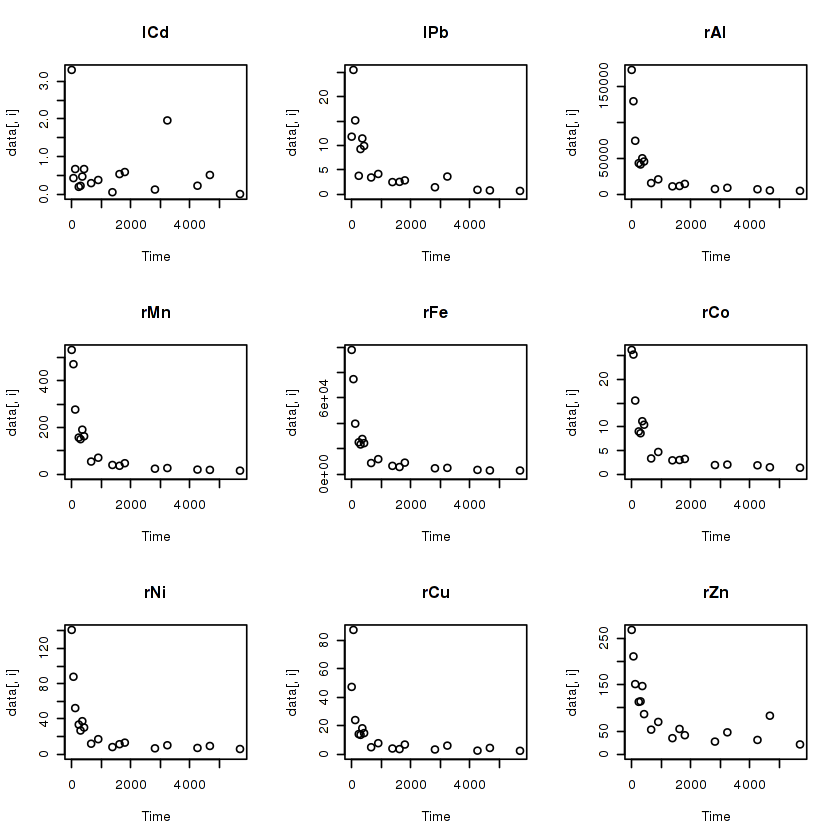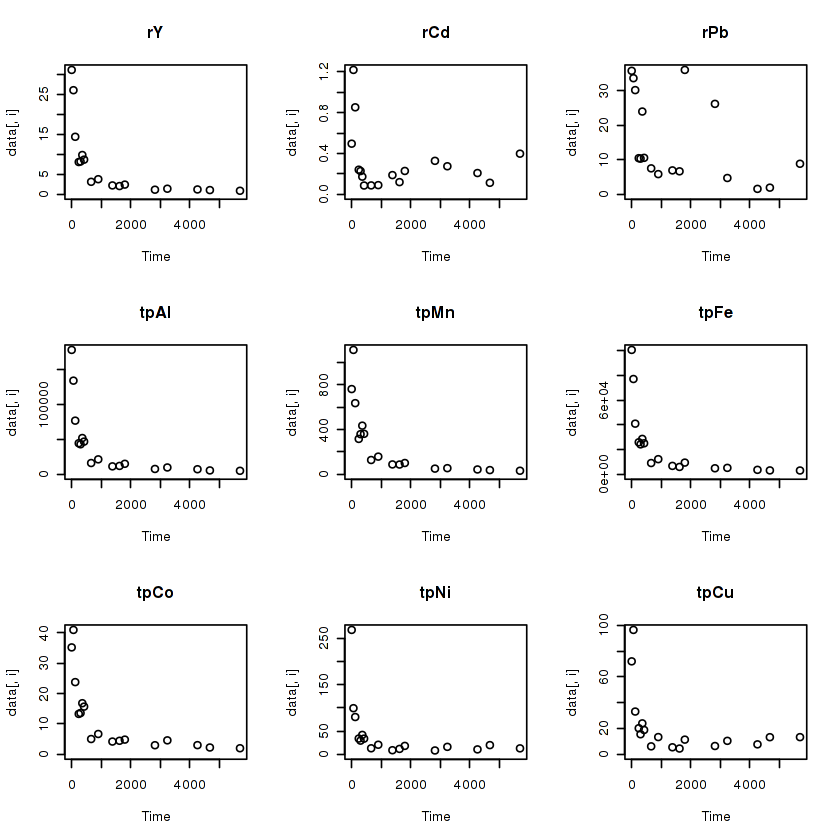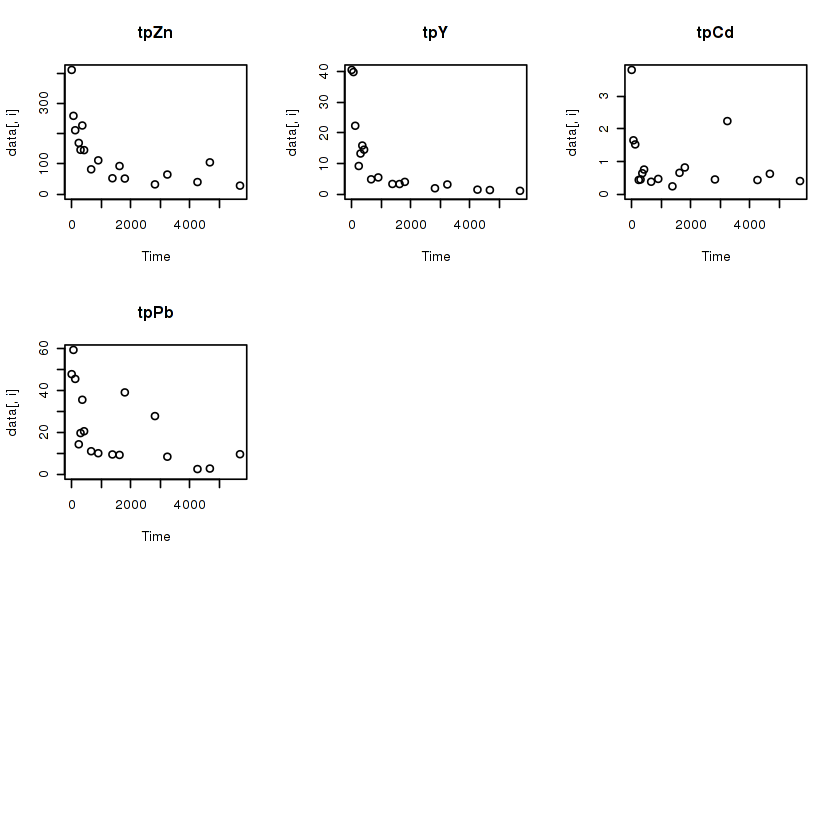In [ ]: